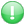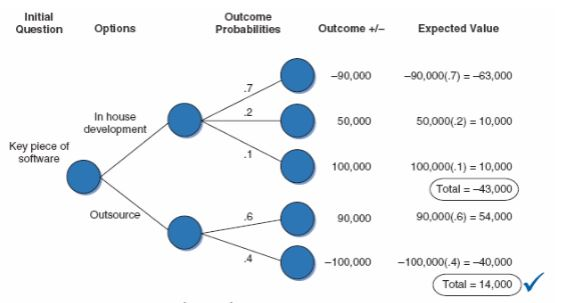Get premium membership and access questions with answers, video lessons as well as revision papers.

# Describe the quantitative risk analysis

Describe the quantitative risk analysis- Much like qualitative risk analysis, quantitative risk analysis attempts to estimate the impact a risk may have on a project as well as the probability
- The key difference between the two is that quantitative analysis is based on mathematical or statistical techniques to model the behavior of a particular risk
- The decision whether to use quantitative or qualitative analysis techniques is made on a project by project basis and can be made on a risk by risk basis
- Techniques include:
(i). Decision trees with expected monetary value analysis
(ii). Simulation (Monte Carlo)

i. Decision Trees and Expected Monetary Value (EMV)
- Decision tree analysis is generally used along with its graphical representation that describes a set of options under consideration along with estimated implications of each option
- The analysis consists of costs, revenues (or benefits), and probabilities for each option path
- The EMV analysis technique is a statistical concept that calculates the average outcome when dealing with unknown future scenarios.ii. Simulation
- Monte Carlo simulation was introduced back in Chapter 6 as an aid in building accurate time estimates for WBS activities
- It can also be used during quantitative risk analysis to simulate the impact a risk may have on project goals
- Based on the probabilities of various outcomes, similar to the decision tree analysis example, the simulation can be run multiple times based on the frequency distribution to determine the expected outcomes with probabilities
- Simulation during the risk management process is generally used to determine cost and schedule outcomes using three point estimates of task durations
francis1897 answered the question on March 14, 2023 at 11:18